# Points Lines Planes Section 1 1 Day 1

• Slides: 9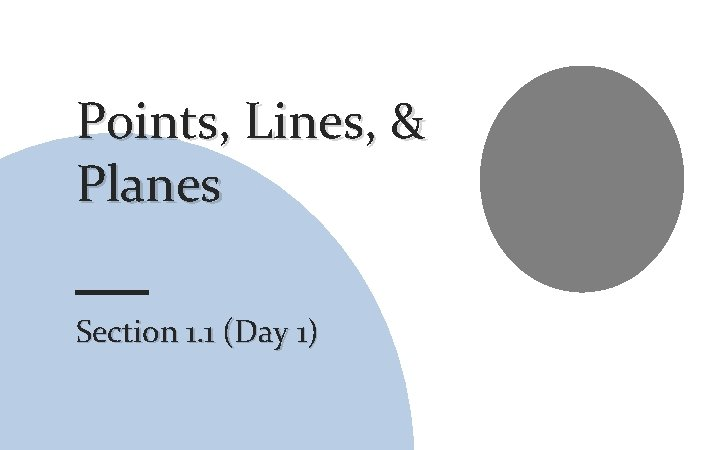Points, Lines, & Planes Section 1. 1 (Day 1)Points Don’t have any actual size. Represented by a dot. Named using ONE capital letter. Point A A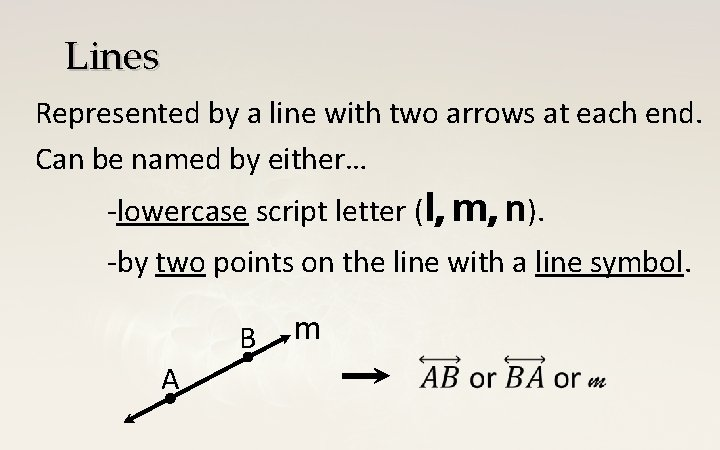Lines Represented by a line with two arrows at each end. Can be named by either… -lowercase script letter (l, m, n). -by two points on the line with a line symbol. B A mCollinear and Non-collinear Points Collinear Points are points on the same line. A C m B D Points A, B, and C are collinear. Point D is non-collinear with points A, B, and C.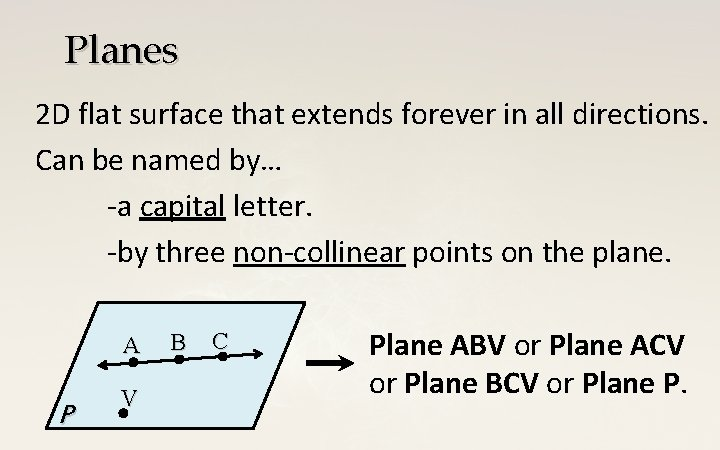Planes 2 D flat surface that extends forever in all directions. Can be named by… -a capital letter. -by three non-collinear points on the plane. A P V B C Plane ABV or Plane ACV or Plane BCV or Plane P.Coplanar Points 3 or more points that lie in the same plane. T A P V B C Points A, B, C, and V are coplanar. Point T is not coplanar with points A, B, C, and V.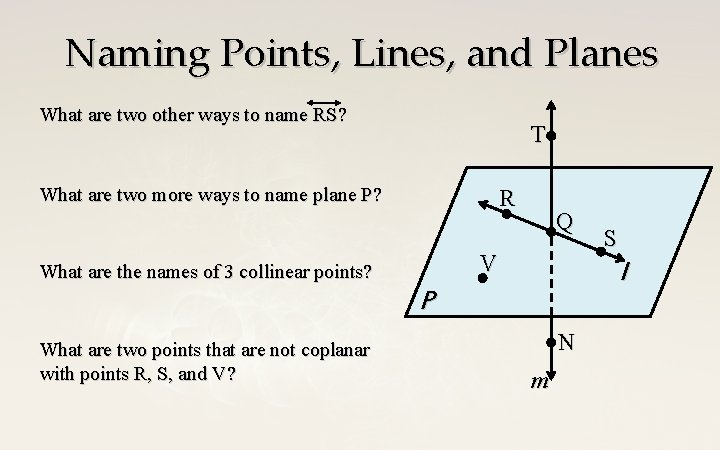Naming Points, Lines, and Planes What are two other ways to name RS? T What are two more ways to name plane P? R Q V What are the names of 3 collinear points? l P What are two points that are not coplanar with points R, S, and V? S N m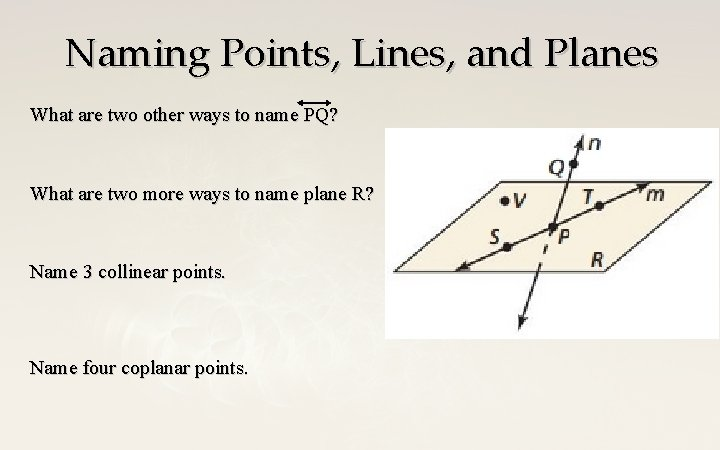Naming Points, Lines, and Planes What are two other ways to name PQ? What are two more ways to name plane R? Name 3 collinear points. Name four coplanar points.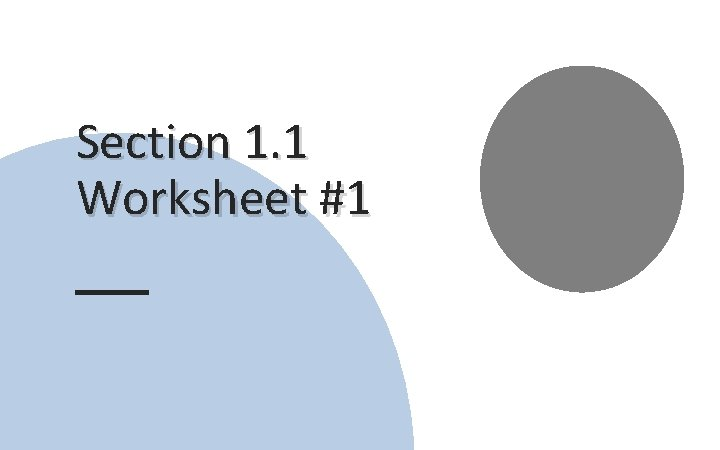Section 1. 1 Worksheet #1